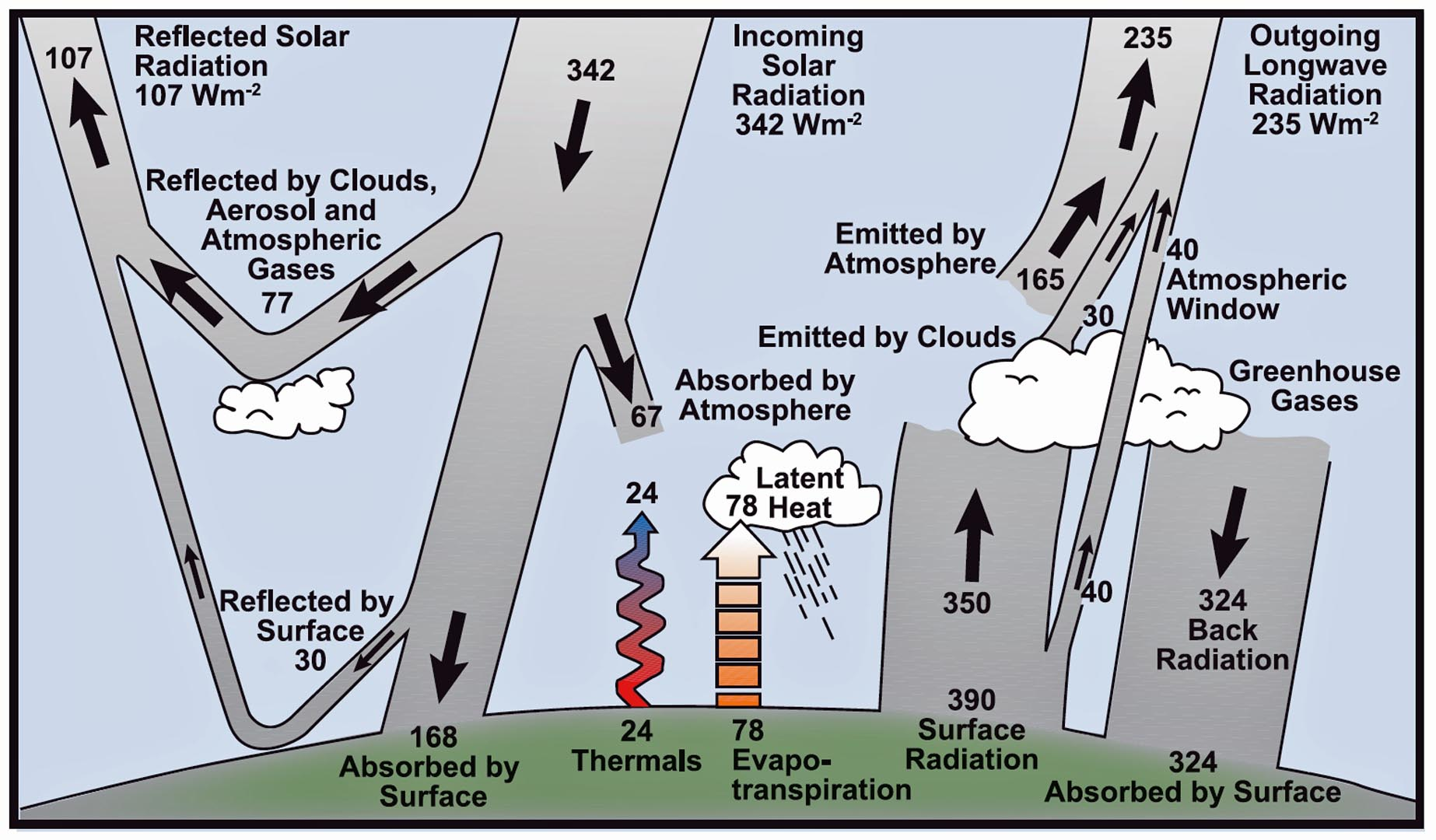Unit B Unit Activity

### Balancing the Earth’s Energy Budget

Objective: The purpose of this activity is to analyze the Earth’s energy budget in greater detail. A mathematical analysis will be done to examine how the Earth’s energy budget is balanced.

Materials Required: Everything that you need for this activity is included in the on-screen components.

Time Required: approximately 30 minutes

The Earth’s energy budget describes the relationship between incoming energy and outgoing energy. On average and over the long term, the Earth is in a state of equilibrium. This means that the Earth has a balanced energy budget with the amount of energy coming in equalled by the amount of energy going out. This balance of “Energy In = Energy Out” should be true at all levels in the Earth system.The Earth’s annual energy budget with numerical values.

The diagram to the right highlights the Earth’s annual energy balance. It is different from the energy budget diagram in lesson B4 in two important respects:

• It gives actual numerical average values for incoming energy and outgoing energy.
• It is more complex and complete in that it explicitly includes the greenhouse effect.

#### PART A: Earth’s Energy Budget at the Top of the Atmosphere

At the top of the atmosphere, the energy coming in from the sun is balanced by the energy reflected back into space and the net outgoing longwave radiation. The equation describing this balance is:

Incoming Solar Radiation = Reflected Solar Radiation + Outgoing Longwave Radiation

1. This equation contains three terms. Examine the energy balance diagram carefully and determine a value for each term.

1. Incoming Solar Radiation =
2. 342 W/m2

3. Reflected Solar Radiation = ?
4. 107 W/m2

5. Outgoing Longwave Radiation = ?
6. 235 W/m2
2. Substitute your values into the equation and verify that it balances.
3.  L.S. (left side of equation) R.S. (right side of equation) Incoming Solar Radiation = 342 W/m2 Reflected Solar Radiation + Outgoing Longwave Radiation = 107 W/m2 + 235 W/m2 = 342 W/m2

L.S. = R.S.

#### PART B: Earth’s Energy Budget at the Earth’s Surface

The energy balance at the Earth’s surface is more complicated because it also involves heating from the greenhouse effect.

1. Use the energy balance diagram to examine the mechanics of the greenhouse effect by determining:

1. How much surface radiation is emitted into the atmosphere?
2. 350 W/m2 is emitted into the atmosphere. (Note: Although the Earth actually emits 390 W/m2, approximately 40 W/m2 travels right through the atmosphere and is lost to outer space. This leaves 350 W/m2 to be absorbed by the atmosphere.)

3. How much of the energy absorbed by greenhouse gases is emitted back to the Earth (i.e. the greenhouse effect)?
4. 324 W/m2 is emitted back to the Earth (indicated as Back Radiation in the diagram).
2. What two other methods allow the Earth to lose some of its absorbed surface radiation?
3. Energy from the Earth’s surface can also be lost through thermals (i.e. through conduction and convection) and through evapotranspiration [i.e. the loss of energy from the ground both by evaporation and by transpiration (the release of water from leaves)].

All incoming energy (i.e. Energy In) at the Earth’s surface in the energy balance diagram is represented by arrows going into the Earth’s surface, while all outgoing energy (i.e. Energy Out) is represented by arrows leaving the Earth’s surface. The complete equation describing this energy balance (i.e. Energy In = Energy Out) at the Earth’s surface is:

Energy Absorbed by Surface + Back Radiation (from Greenhouse Effect)
= Surface Radiation + Evapotranspiration + Thermals

1. Use values from the energy balance diagram to confirm this equation. (Note: The “Surface Radiation” term refers to net surface radiation.)
2.  L.S. (left side of equation) R.S. (right side of equation) Energy Absorbed by Surface + Back Radiation (from greenhouse effect) = 168 W/m2 + 324 W/m2 = 492 W/m2 Surface Radiation + Evapotranspiration + Thermals = 390 W/m2 + 78 W/m2 + 24 W/m2 = 492 W/m2

L.S. = R.S.

#### PART C: Earth’s Energy Budget in the Atmosphere

The most complicated relationship exists in the atmosphere, in part due to the greenhouse effect. Energy from sunlight absorbed by the atmosphere and energy from the Earth’s surface must be balanced by outgoing energy emitted from the atmosphere. The complete equation describing this energy balance in the atmosphere is given by:

Sunlight Absorbed by Atmosphere + Thermals + Evapotranspiration + Surface Radiation from Earth (into atmosphere) = Energy Emitted Back to Ground (i.e. Back Radiation) + Energy Emitted by Clouds + Energy Emitted by Atmosphere

1. Use values from the energy balance diagram to confirm this equation.
2.  L.S. (left side of equation) R.S. (right side of equation) Sunlight Absorbed by Atmosphere + Thermals + Evapotranspiration + Surface Radiation from Earth (into atmosphere) = 67 W/m2 + 24 W/m2 + 78 W/m2 + 350 W/m2 = 519 W/m2 Energy Emitted Back to Ground (i.e. Back Radiation) + Energy Emitted by Clouds + Energy Emitted by Atmosphere = 324 W/m2 + 30 W/m2 + 165 W/m2 = 519 W/m2

L.S. = R.S.